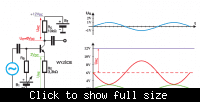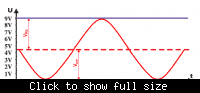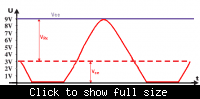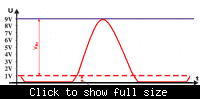# What exactly is maximum symmetrical swing?

NewtonianAlch
I see this term quite a lot for BJT's and I can't quite figure out exactly what it is, although I have some idea based on a bit of reading from different sources.

Is there such a thing as unsymmetrical swing, and what would that be?

## Answers and Replies

Jony130
Try to analysis this exampleVcc = 12V; Vc = 6V (red plot ); Ve = 2V (green plot) ; Vinput = 1V (blue plot).

To make sure that BJT work in the linear region and to achieve a large voltage swing you need to set the bias point (voltage at collector) equal to 0.5Vcc (and this assumption automatically ensures that the BJT will work in the active region).

And this imagines show why we need Vce = 0.5*Vcc

Vce=4.5V maximum symmetrical swing +/- 4.5VVce=3V maximum symmetrical swing +3V and -3VVce=1V
maximum symmetrical swing +1V and -1VSo to amplifier our input signal waveform ( positive half and negative half) without distortion we need to set dc bias point at 0.5Vcc.

yungman
Even if there is no clipping, you can have distorted waveform that is not symmetrical. For a resistor load common emitter stage, even harmonics are quite high and if you look at the wave form, one side is fatter and the peak amplitude is a little lower than the other side. It is progressively more prominent as the output swing increases.

Studiot
I like the pics and explanation, Jony!NewtonianAlch
Ah...thanks a lot for those pictures I see now what happens when the bias point is not half of Vcc.

That was very helpful.